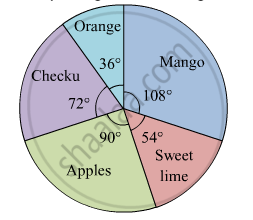# The Following Table Shows the Percentages of Demands for Different Fruits Registered with a Fruit Vendor. Show the Information by a Pie Diagram. - Algebra

Diagram

The following table shows the percentages of demands for different fruits registered with a fruit vendor. Show the information by a pie diagram.

 Fruits Mango Sweet lime Apples Cheeku Oranges Percentages of demand 30 15 25 20 10

#### Solution

The measures of central angles are given  in the table.

 Fruits Percentages of demand Central Angle Mango 30 $\frac{30}{100} \times 360 = 108^\circ$ Sweet lime 15 $\frac{15}{100} \times 360 = 54^\circ$ Apples 25 $\frac{25}{100} \times 360 = 90^\circ$ Cheeku 20 $\frac{20}{100} \times 360 = 72^\circ$ Oranges 10 $\frac{10}{100} \times 360 = 36^\circ$ Total 100

The pie digram showing the above data is given below:Concept: Concept of Pie Graph (Or a Circle-graph)
Is there an error in this question or solution?

#### APPEARS IN

Balbharati Mathematics 1 Algebra 10th Standard SSC Maharashtra State Board
Chapter 6 Statistics
Practice Set 6.6 | Q 4 | Page 164